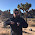Wednesday, February 24, 2016

Information equilibrium and transistors (updated)

This is a bit of an aside; I'm going to create an additional model that can be related to information equilibrium that may provide a source of helpful analogies. Consider a transistor with emitter current $i$ and base voltage $V$ and consider the information equilibrium relationship $V \rightleftarrows i$ (base voltage is the information source and emitter current is the information destination):

$$\frac{dV}{di} = k \frac{V}{i}$$
with slow changes in the voltage relative to current (current adjusts faster to changes in voltage than voltage adjusts to changes in current). That gives us the "partial equilibrium" (in economics parlance) solution

$$k V_{0} \log \frac{i}{i_{ref}} = V - V_{ref}$$

take $V \gg V_{ref}$ and rearrange to obtain

$$i = i_{ref} \exp \frac{V}{k V_{0}}$$

This is the Ebers-Moll model of a transistor in the forward region, acting as an amplifier.

I brought this up because Cesar Hidalgo used a transistor metaphor in Why Information Grows:
Now consider that we can push [a chemical] system to one of [its] steady states by changing the concentration of inputs ... Such a system will be “computing,” since it will be generating outputs that are conditional on the inputs it is ingesting. It would be a chemical transistor.
Information equilibrium relationships can represent supply and demand (information flowing between them ... see the paper). We add a new metaphor borrowed from Hidalgo: information flowing between the base voltage and the emitter current in a transistor. In this case, traveling along supply and demand curves can be seen as the linear region of an amplifier which faithfully reproduces the information in the weak signal at the output.

In the example, voltage is the demand for electrons and current is the supply of electrons. Our partial equilibrium solution represents movements along a demand curve. Note that the abstract price in this example is the (effective) resistance $R$  -- the RHS of the first equation is half of Ohm's law.

$$P \equiv \frac{dV}{di} = k \frac{V}{i} \propto R$$
such that we get the price relationship of  a demand curve (increase base voltage $V$ and you get a fall in the price/resistance):

$$R = P = \frac{k V_{0}}{i_{ref}} \exp \left( - \frac{V}{k V_{0}} \right)$$

Changes in temperature (which change the thermal voltage that maps to $V_{0}$, see 25 Feb update below) are shifts of the demand curve.

Footnotes

 Note this is the effective resistance: assuming a voltage applied at the base produces the amplified current at the emitter. It's not the resistance of the current across the transistor (collector-emitter). For a large enough voltage, the effective resistance can go close to zero ... but this isn't a superconductor. The current is coming from the other terminal of the transistor.

[Updated 26 Feb 2016]

...

PS Sorry for the tenseness of the post. It was composed one finger tap at a time on an iPad. [Updated 26 Feb 2016 to be a little less terse.]

...

Update 25 Feb 2016

I have extended the above results to include the Early effect (Early is a person's name). The Early effect can be derived through a charge conservation argument (depolarization across the PN boundaries). I'll derive it via information equilibrium below. But there is a question: why should a physical process know about conserving information? The answer is in the question. Information equilibrium is an information conservation argument -- information carried by those charges. In places where we expect the transistor to operate without information loss (the forward active region), then we should expect charge conservation to produce results consistent with information equilibrium.

Here's the derivation and a diagram to go along with it:

In nice $\LaTeX$ form

$$i \approx i_{ref} \exp \frac{V}{k V_{0}} \left(1 - \frac{V_{ref}}{k V_{0}} \right)$$

or in terms of the base-emitter voltage (BE), collector-emitter voltage (CE), the Early voltage (A) and the thermal voltage (T):

$$i \approx i_{ref} \exp \frac{V_{BE}}{k V_{T}} \left(1 + \frac{V_{CE}}{k V_{A}} \right)$$

Interestingly, we can add the IP3 point (well, not exactly, but its IV analog instead of power) to get a good example of how ideal and non-ideal information transfer describe the system at various times. An amplifier is operating successfully when the information in is equal to the information out ... just louder. This happens in the "linear" (log-linear) region after the saturation, but before the nonlinearities (compression) described by e.g. the "IP3 point" kick in. Inside this region, information equilibrium is a good approximation:

But in the other regions we should expect less current than an ideal (information) amplifier because I(i) < I(V). And that is generally what is observed.

...

PS The onset of current at some small voltage at the base is what gives a transistor its switch-like properties.

1.Great! I need a new analogy to work with.

1.Looks like there will be a bit more to this ... the Early effect can be added (it's a consequence of charge conservation, which makes sense as charge is carrying the information).

2.I'm looking forward to your scattering parameters model with pole and zero formulated equilibria.

Henry

1.I don't expect information equilibrium to apply except as a first order approximation ... Sort of like how a fermi gas model doesn't apply to semiconductor band structure.

Comments are welcome. Please see the Moderation and comment policy.

Also, try to avoid the use of dollar signs as they interfere with my setup of mathjax. I left it set up that way because I think this is funny for an economics blog. You can use € or £ instead.